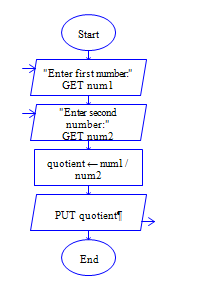# Python Integer Division

Python program to get two integer numbers, divide both the integers and display the Integer quotient.

Sample Input 1:

10 3

Sample Output 1:

3

Sample Input 2:

29 2

Sample Output 2:

14

#### Flow Chart Design#### Program or Solution

``` num1=int(input("Enter First number:")) num2=int(input("Enter second number:")) quotient=num1//num2 print(quotient) ```

#### Program Explanation

Read two inputs using input() method.

Convert it into integer by int() method.

Divide using // operator, which gives integer quotient.